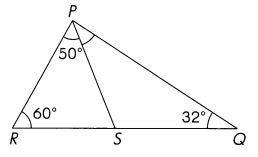# Math in Focus Grade 5 Chapter 13 Practice 2 Answer Key Measures of Angles of a Triangle

This handy Math in Focus Grade 5 Workbook Answer Key Chapter 13 Practice 2 Measures of Angles of a Triangle provides detailed solutions for the textbook questions.

## Math in Focus Grade 5 Chapter 13 Practice 2 Answer Key Measures of Angles of a Triangle

Complete.

Question 1.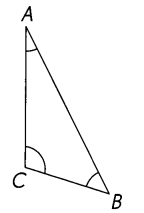m∠A + m∠B + m∠C = ____
m∠A + m∠B + m∠C =180°
Explanation:
The property of a triangle is : The sum of all internal angles of a triangle is always equal to 180°.
m∠A + m∠B + m∠C = 180°

Question 2.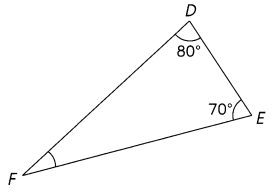80° + 70° + m∠F = ___
m∠F = ___
80° + 70° + m∠F = 180°
m∠F = 30°
Explanation:
The property of a triangle is : The sum of all internal angles of a triangle is always equal to 180°.
80° + 70° + m∠F = 180°
m∠F = 180°- 80° + 70° = 30°

Measure the angles of the triangle. Then fill in the blanks.

Question 3.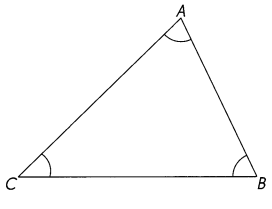m∠A = ___
m∠B = ___
m∠C = ____
m∠A + m∠B + m∠C = __ + ___ + ___
= ____
The sum of the angle measures in the triangle is ___
m∠C = 40°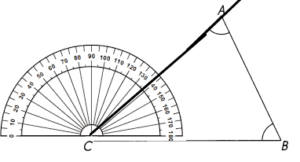m∠B = 60°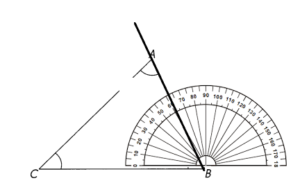The sum of angles in a triangle are 180°
40 + 60 = 100
180 – 100 = 80°
m∠A = 80°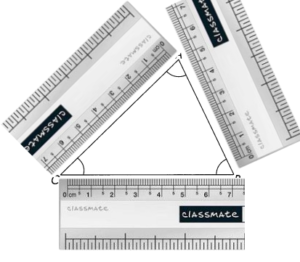m∠A = 80°
m∠B = 40°
m∠C = 60°
m∠A + m∠B + m∠C = 80°+ 40°+ 60° = 180°

These triangles are not drawn to scale. Find the unknown angle measures.

Question 4.
Find the measure of ∠B.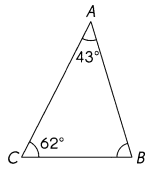m∠B = 75°
Explanation:
The property of a triangle is : The sum of all internal angles of a triangle is always equal to 180°.
43° + 62° + m∠B = 180°
m∠B = 180° – 43° + 62° = 75°

Question 5.
Find the measure of ∠D.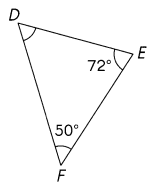m∠D = 68°
Explanation:
The property of a triangle is : The sum of all internal angles of a triangle is always equal to 180°.
72° + 50° + m∠D = 180°
m∠D = 180° – 72° + 50° = 68°

Question 6.
Find the measure of ∠H.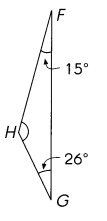m∠H  = 139°
Explanation:
The property of a triangle is : The sum of all internal angles of a triangle is always equal to 180°.
15° + 26° + m∠H = 180°
m∠H = 180° – 15° + 26° = 139°

Question 7.
Find the measure of ∠QPS.